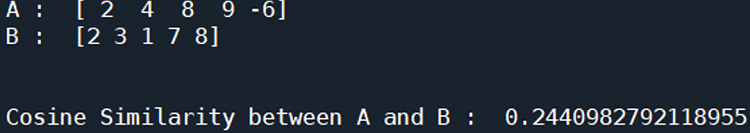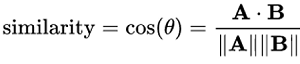# Cosine Similarity between two vectors | Linear Algebra using Python

Linear Algebra using Python | Cosine Similarity between two vectors: Here, we are going to learn about the cosine similarity between two vectors and its implementation in Python.
Submitted by Anuj Singh, on June 20, 2020

Prerequisite:Cosine similarity is a metric used to measure how similar the vectors are irrespective of their size. Mathematically, it is a measure of the cosine of the angle between two vectors in a multi-dimensional space. The cosine similarity is advantageous because even if the two similar vectors are far apart by the Euclidean distance, chances are they may still be oriented closer together. The smaller the angle, the higher the cosine similarity.## Python code for cosine similarity between two vectors

```# Linear Algebra Learning Sequence
# Cosine Similarity

import numpy as np

a = np.array([2, 4, 8, 9, -6])
b = np.array([2, 3, 1, 7, 8])

ma = np.linalg.norm(a)
mb = np.linalg.norm(b)

print('A : ', a)
print('B : ', b)

# Cosine Similarity
sim = (np.matmul(a,b))/(ma*mb)

print('\n\nCosine Similarity between A and B : ', sim)
```

Output:

```A :  [ 2  4  8  9 -6]
B :  [2 3 1 7 8]

Cosine Similarity between A and B :  0.2440982792118955
```

Preparation

What's New

Top Interview Coding Problems/Challenges!